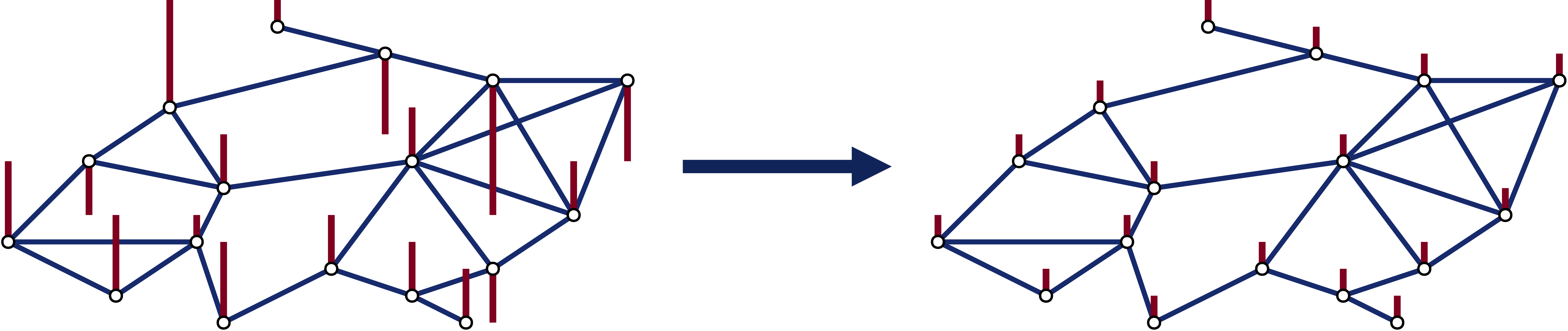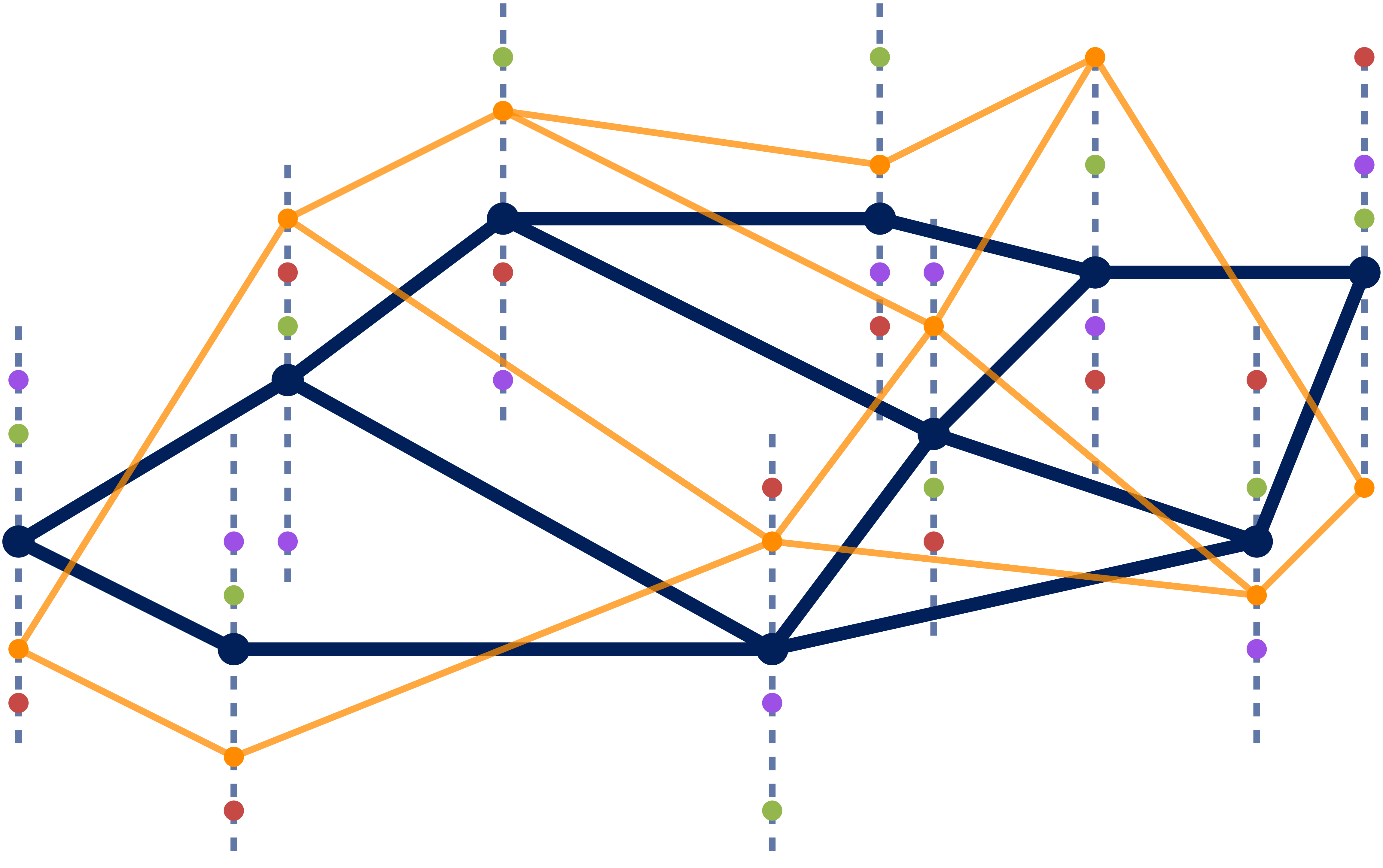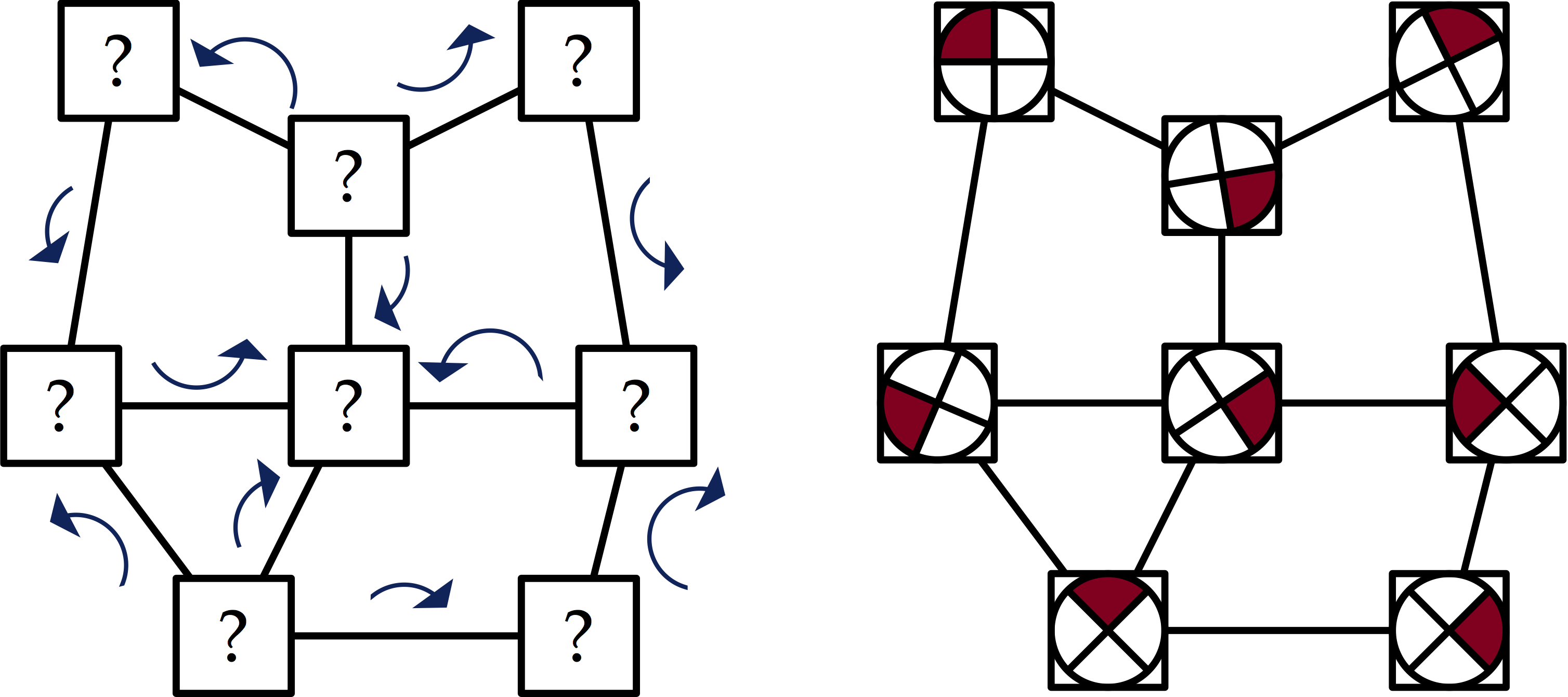I study aspects of data and systems using cellular sheaves, a tool inspired by algebraic topology. In particular, I am interested in topologically-inspired approaches in areas like these:

### Network ConsensusA key insight from the systems engineering community in recent years has been the fact that simple algorithms can produce sophisticated behaviors in networks of agents. One prominent example is the theory of network consensus. A collection of agents with varied initial states, can, by only communicating with their neighbors, converge to consensus on the global average of the initial states. Lifting this insight to cellular sheaves gives an iterative algorithm that converges to a global section of the sheaf given any initial condition.

Consensus-type algorithms can also be used as a component in bigger systems, in particular for problems like distributed optimization. There are natural consensus and optimization problems over cellular sheaves, and sheaf Laplacians are a natural tool for constructing distributed algorithms for their solution.

This algorithm gives a potential way to reduce communications costs when running consensus algorithms with high-dimensional data. Rather than using the constant sheaf, which would require each agent to communicate its entire state vector to each neighbor at every step, we can use an approximation to the constant sheaf, which has the same space of global sections, but lower communications requirements. Current work includes finding efficient ways to construct good approximations to the constant sheaf.

### Understanding NetworksNetwork science has produced remarkable insights using simple models of connectivity: two nodes are either connected or not. A recent trend broadens this analysis to more complex models, like signed graphs or multilayer networks. Cellular sheaves (particularly through their Laplacians) offer another avenue for analysis of not just whether two nodes are connected, but how they are connected.

A simple example of the way in which sheaves on graphs can elucidate deeper structure in networks is when constructing a network from data. If we have signals associated with the nodes of a network, we might construct a network assuming that edges exist between nodes that tend to have nearly equal values of signals. But this can miss structure in the data: what if signals at two nodes tends to be nearly opposite, or more generally to have some linear relationship? Rather than learning a graph, we can learn a sheaf to extract these more subtle relationships from the data.

### SynchronizationSynchronization is a data analysis problem where we estimate some unknown parameters from knowledge of the pairwise relationships between some of those parameters. The canonical example of this is the $$O(n)$$ synchronization problem, where the relative orientations of some set of objects are known and we want to obtain a global orientation frame. Synchronization techniques have applications to problems in cryo-electron microscopy, as studied by Amit Singer and Afonso Bandeira. The topological structure underlying synchronization problems has been described in terms of of Čech cohomology and flat vector bundles. However, a more general topological formulation is as follows:

Synchronization is the problem of finding a global section of a given cellular sheaf.

I am working to build a theory of synchronization problems in the language of cellular sheaves, with hopes of producing insights and algorithms.

### Manifold Learning

Manifold learning is concerned with finding nonlinear low-dimensional structure in high-dimensional data. Many approaches exist, with different goals and ideas. Almost none of them incorporate topological information. A key part of the structure of a manifold is the various sheaves it carries–of functions, of vector fields, of differential forms–and making that structure explicit in dimensionality reduction and manifold learning is a promising idea.

### Spectral Methods

Topological approaches to problems in data analysis and systems design often founder on the noise and error encountered in the real world. Topological conditions such as the existence of global sections of a sheaf are not robust with respect to perturbations of the underlying structure. Applying topology to real-world problems requires the development of robust and approximate techniques. One approach to approximate topology is persistence theory, which has a strong theoretical basis and nearly two decades of research activity. Another approach involves the extension of spectral graph theory to higher-dimensional simplicial complexes. Since much of my work involves sheaves on simplicial complexes, I am developing a spectral theory of cellular sheaves, inspired by spectral graph theory, that helps answer questions about problems that can be phrased in terms of cellular sheaves.